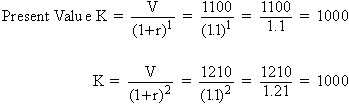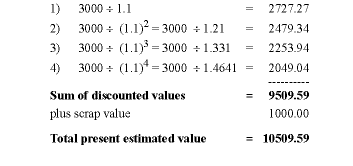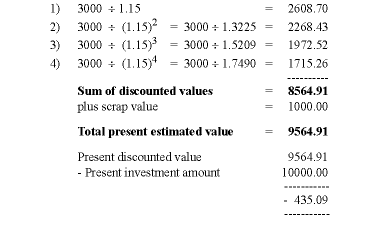Home MonkeyNotes Printable Notes Digital Library Study Guides Study Smart Parents Tips College Planning Test Prep Fun Zone Help / FAQ How to Cite New Title Request

15.4 Investment Decisions

(A) Discounting: One important but difficult part in the process of transactions in the capital market is about appropriate investment decisions. How does one decide whether a certain type of investment will be profitable or not? Let us continue with our earlier example:

A lender who gives a loan of \$1000 receives \$1100 after a year and \$1210 after two years when the rate of interest is 10 percent. These future values of present lending are determined by the compounding process.

Future Value V = K (1 + r)1 = 1000(1 + 0 .10) 1 = 1000(1 .1) = 1100

V = K (1 + r)2

= 1000(1 .10)2 = 1000 (1.21) = 1210

In this example, K is the present lending value and V, the future value. Since we already know the expected future income, to arrive at its equivalent present value, we need to reverse the process or discount the future values at the rate of the current interest rate.(B) Investment Decisions: There is a similar method employed for discounting future streams of income which is applicable in making appropriate investment decisions. Let us assume that a taxi driver wants to purchase his own car and to use it as a taxi for earning regular income. Suppose the present market price of the taxi cab is \$1000. The present rate of interest (discount) is 10 percent. The life of the taxi is likely to be four years. During these years the taxi driver expects to earn an average annual income of \$3000. At the end of the fourth year the vehicle cannot any more be used as a taxi but can be sold out as scrap at a value of \$1000. Keeping this in mind the driver has to decide if it will be worth purchasing the car. At a glance it appears that the taxi will bring a total income of \$12000 (3000 ´ 4) plus scrap value of \$1000. The sum of \$13000 as future income on the present investment is attractive. However, other factors must also be considered. The expected income streams in the future have to be discounted at the rate of 10 percent because the market rate of interest acts as an opportunity cost. The taxi owner could have earned this much by depositing \$10000 with the bank if he were to possess such resources. Otherwise he could have borrowed \$10000 from the bank and promised to pay interest charges. The present discounted value of these expected incomes would be as follows:In the present case the discounted value of 10509.59 exceeds the investment value of \$10,000 by \$509.59. Therefore the investment decision is profitable. If the present value were lower than the total investment, and subsequently, if the difference were negative then the investment decision would not have been profitable.

Let us assume that the market rate of interest is as high as 15 percent but retain the initial investment amount of \$10,000 and the final scrap value of \$1000. The present discount value of the capital asset will be negative and it will not be worth while to invest in it. Discounted values of the expected incomes in this case are as follows:Since the net addition to the income is negative, an investment decision will not be profitable.

Let us briefly summarize the two cases.

Case 1: Profitable          Case 2: Net Profitable

Initial Investment \$10000 Initial Investment \$10,000

Rate of Interest   10% ;      Rate of Interest 15%

Scrap value \$1000           Scrap value \$1000

 Sr. No. Expected Annual Income Discounted Present Value Expected Annual Income Discounted Present Value 1 3000 2727.27 3000 2608.7 2 3000 2479.34 3000 2268.43 3 3000 2253.94 3000 1972.52 4 3000 2049.04 3000 1715.26 1000 2040.04 1000 10509.59 9564.91 -10000 -10000 Net Value +509.59 Net Value -435.09

(C) Practical Difficulties: A decision regarding investment is a complex and difficult activity. To compute discounted values and to compare them with expected income is a formidable task. The method explained above is quite similar to the Keynesian concept of Marginal Efficiency of Capital (MEC). In actual decision making there are further risks involved. We have assumed fixed average future expected returns. We have also assumed uniform constant rate of interest. Both these expectations are likely to go wrong. Such conditions of uncertainty take away the possibility of accurate investment decision making. The only conclusion we can draw is that investment decisions are likely to be more profitable at a relatively lower rate of interest and less profitable at a relatively higher rate of interest. Therefore a lower rate of interest which simultaneously falls is more favorable to investment activity.

**********

Index

15.1 - Basic Concepts
15.2 - Productivity of Capital
15.3 - Market Rate of Interest
15.4 - Investment Decisions

 Search: All Products Books Popular Music Classical Music Video DVD Toys & Games Electronics Software Tools & Hardware Outdoor Living Kitchen & Housewares Camera & Photo Cell Phones Keywords: